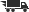Close

# Introduction to Matlab for Engineers and Scientists

## by Delores M. Etter

• ISBN: 9780135197035
• ISBN10: 0135197031

# Introduction to Matlab for Engineers and Scientists

## by Delores M. Etter

• List Price: \$42.40
• Binding: Paperback
• Publisher: Prentice Hall
• Publish date: 04/01/1996
• ISBN: 9780135197035
• ISBN10: 0135197031
used \$2.80
You save: 93%
new \$200.08
Description: 1. An Introduction to Engineering Problem Solving. Grand challenges. Computing systems. An engineering problemsolving methodology. 2. MATLAB Environment. Characteristics of the MATLAB environment. Scalars, vectors, and matrices. Scalar and array operations. Special values and special matrices. Additional plotting capabilities. Problem solving applied: Velocity computations. 3. MATLAB Functions. Math functions and trigonometric functions. Data analysis functions. Random numbers. Problem solving applied: Flight simulator. Selection statements and selection functions. User-written functions. 4. Matrix Computations. Matrix operations and functions. Solutions to systems of linear equations. 5. Symbolic Mathematics. Symbolic algebra. Equation solving. Differentiation and integration. 6. Numerical Techniques. Interpolation. Curve fitting. Numerical integration. Numerical differentiation. Complete Solutions to Practice! Problems. Index.
Expand description
 Seller: Seattle Goodwill ★★★★★ ★★★★★ Location: Seattle, WA Condition: GoodMay have some shelf-wear due to normal use. May have some shelf-wear due to normal use. Price: \$2.80 Comments: May have some shelf-wear due to normal use. May have some shelf-wear due to normal use.
 Seller: One Stop Shop Bookstore ★★★★★ ★★★★★ Location: Memphis, TN Condition: GoodPrice: \$23.57 Comments: